MATH 42 helps students to understand math and solve their homework

APP Information

 Download Version 3.3.24 (62) Apk Size 27.31 MB App Developer Cogeon Malware Check UNKNOWN Install on Android 5.1 and up App Package com.math42solutions.apk MD5 26915946f21ac24181e4ed6db7c03cf7 Rate 4.33 Website http://math-42.com### App Description

```MATH 42 helps over 2.200.000 middle-school, high-school and college students, to solve their math problems and get better grades at the fraction of the cost of a private tutor. MATH 42 helps with (1) intelligent approaches to the solution, (2) step-by-step solutions of their problems (3) an Assessment Center.

MATH 42 is a trusted mathematical resource around the world and the numbers prove it: Over 2.2 million downloads, used on over 450.000 tablets in schools, in cooperation with the biggest educational publisher in Germany (Klett).

FEATURES

• Intuitive entry of formulas
• Intelligent suggestions on how to approach a problem (unique worldwide)
• Detailed step-by-step solutions, that adapt to a student’s need (unique worldwide)
• Extensive corresponding mathematical explanations with examples
• Interactive graphs, that visualize problems
• Automatically generated and curated assessment, that enables and facilitates rapid progress
• Instant-Calculator, that computes during the entry

ASSESSMENT CENTER

• Exercises — subdivided by topic and level
• Training mode — afterwards, each problem can be analyzed step-by-step
• Test mode — enabling the identification of strengths and weaknesses
• Test results and statistics show progress over time
• Tests and exercises were created in cooperation with experienced pedagogues and private tutors

MATHEMATICAL FEATURES

Term Transformation
• Simplyfing and expanding
• Factorization (Factoring out, application of the binomial formulas)
• Transforming powers and roots
• Adding fractions, multiplying fractions, reducing fractions, expanding fractions
• Computing the gcd and the lcm
• Trigonometric transformations (Is being enhanced)
• Logarithmic transformations (Is being enhanced)

Functions
• Roots
• Y-axis intersection
• Domain of definition
• Extremes (Maximums, Minimums)
• Inflexion points
• Curve sketching
• Derivation
• Fundamental derivatives,
• Factor rule
• Sum rule
• Product rule
• Quotient rule
• Chain rule
• Integration
• Fundamental integrals,
• Integration by substitution
• Integration by parts
• Partial fraction decomposition

Equations
• Linear equations
• Special equations of higher degree
• Linear systems of equations
• Non-linear system of equations

Matrices
• Multiplying matrices
• Transposing matrices
• Inverting matrices
• Determinants
• Characteristic polynomials
• Eigenvalues
• Eigenspaces
• Kernels
• Ranks
• Cross-product
• Gaussian elimination

TECHNOLOGY

• MATH 42 works without templates or pre-calculated solutions
• MATH 42 solves all problems on the fly, like a teacher at the board
• MATH 42 is a real symbolic algebra system
• MATH 42 bases on artificial intelligence
• MATH 42 is a completely self-developed system
```

### App ChangeLog

• MATH 42 — your digital private math tutor — is completely free now!

### More Android Apps to Consider

1.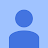Jakob Holzner

It always says:" no response from our servers. Please be sure to have an internet connection" Not usable.

2.Germano Migliastro

Unable to contact server. Update one year later: The problem persists. It's a shame because before being acquired by Chegg it was a great app, did they do it to kill it? Update 15 minutes later: Mistery unveiled. They did it. They bought this app in order to kill it and let their own app gain downloads. Resetting my review to 5 stars, i suggest to not download the other Chegg math app and try different solutions, there probably are many valueble apps. Sad that this had to die. RIP my good friend

3.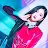stan loona and weeekly!

it's great, I've used it for years and its detailed explanation helped me a lot when I started learning calculus in high school. There could be some improvements though, you can still work around them but eh, its kind of bothersome (like a few improvements with trigonometric functions) and also I noticed how some solutions arent simplified and it gives you a long answer (like the derivative of (x^4/4)ln(x)-(x^4/16) )

4.Princess Muchindu

Math 42 is an awesome app,its helped me understand some of the most complex math problems,i just wish there was a little bit more detail on the answers, for much easier understanding ,thats why i gave it four stars,but all in all u guys are best thank u.

5.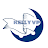Kelly VP

This app is really awesome, the step by step calculation of an equation the another level, however the theoretical part can be improved by adding more explanations. Thanks!

6.Mar Vin

Most of the exercises in our Math Schoolbock don't have any explanation, so I had the problem to understand the way pf doing tht exercises. You guys help me to understand it on a really detailed and cool way. Thank you very much!

7.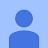It's perfect, helped me a lot. And yes you have to log in but where is the problem? It shows you the correct solution and how you can solve it.

8.It does not work when want to write squer 3 or squer 4, but yeah in squer 2 it works and it is good app if it is possible to fixe it could be so good and i wanted to notice about it to my other friends too, but yeah.....

9.micheal atoyebi

The app was doing well until lately when it started failing. Let the admin rectify the issues. When one input any question, it doesn't solve it

10.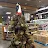Alex Frentz

shockingly awesome in both ambition and (as far as I can tell) implementation. please keep me posted. -- professional in the math education sector